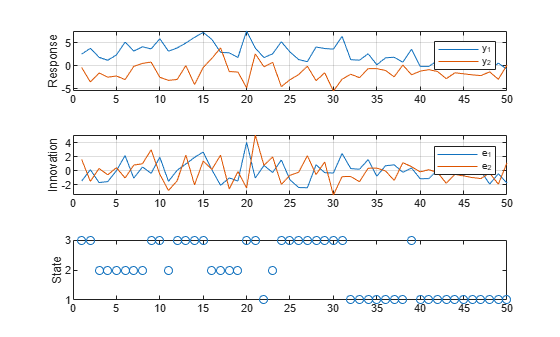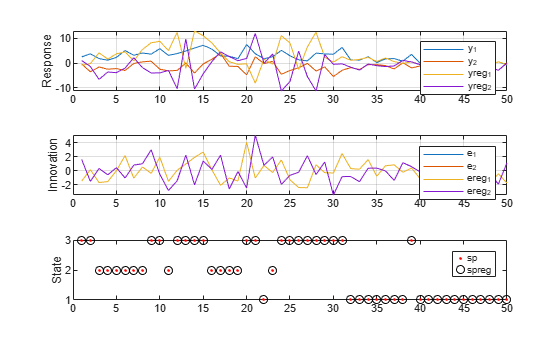# Simulate Multivariate Markov-Switching Dynamic Regression Model

This example shows how to generate random response and state paths from a two-state Markov-switching dynamic regression model.

Consider the response processes ${\mathit{y}}_{1\mathit{t}}$ and ${\mathit{y}}_{2\mathit{t}}$ that switch between three states, governed by the latent process ${\mathit{s}}_{\mathit{t}}$ with this observed transition matrix:

`$P=\left[\begin{array}{ccc}10& 1& 1\\ 1& 10& 1\\ 1& 1& 10\end{array}\right].$`

Suppose that ${y}_{t}={\left[\begin{array}{cc}{y}_{1t}& {y}_{2t}\end{array}\right]}^{\prime }$ is a VARX model in each state:

`${y}_{t}=\left\{\begin{array}{ll}\left[\begin{array}{c}1\\ -1\end{array}\right]+\left[\begin{array}{c}1\\ -1\end{array}\right]{x}_{1t}+{\epsilon }_{1t}& ;{s}_{t}=1\\ \left[\begin{array}{c}2\\ -2\end{array}\right]+\left[\begin{array}{cc}0.5& 0.1\\ 0.5& 0.5\end{array}\right]{y}_{t-1}+\left[\begin{array}{cc}2& 2\\ -2& -2\end{array}\right]\left[\begin{array}{c}{x}_{1t}\\ {x}_{2t}\end{array}\right]+{\epsilon }_{2t}& ;{s}_{t}=2\\ \left[\begin{array}{c}3\\ -3\end{array}\right]+\left[\begin{array}{cc}0.25& 0\\ 0& 0\end{array}\right]{y}_{t-1}+\left[\begin{array}{cc}0& 0\\ 0.25& 0\end{array}\right]{y}_{t-2}+\left[\begin{array}{ccc}3& 3& 3\\ -3& -3& -3\end{array}\right]\left[\begin{array}{c}{x}_{1t}\\ {x}_{2t}\\ {x}_{3t}\end{array}\right]+{\epsilon }_{3t}& ;{s}_{t}=3,\end{array}$`

where, for j = 1,2,3, ${\epsilon }_{\mathrm{jt}}$ is Gaussian with mean 0 and covariance matrix

`${\Sigma }_{j}=j\left[\begin{array}{cc}1& -0.1\\ -0.1& 1\end{array}\right].$`

### Create Fully Specified Model

Create the Markov-switching dynamic regression model that describes ${\mathit{y}}_{\mathit{t}}$ and ${\mathit{s}}_{\mathit{t}}$.

```% Switching mechanism P = [10 1 1; 1 10 1; 1 1 10]; mc = dtmc(P); % VAR submodels C1 = [1;-1]; C2 = [2;-2]; C3 = [3;-3]; AR1 = {}; AR2 = {[0.5 0.1; 0.5 0.5]}; AR3 = {[0.25 0; 0 0] [0 0; 0.25 0]}; Beta1 = [1; -1]; Beta2 = [2 2;-2 -2]; Beta3 = [3 3 3;-3 -3 -3]; Sigma1 = [1 -0.1; -0.1 1]; Sigma2 = [2 -0.2; -0.2 2]; Sigma3 = [3 -0.3; -0.3 3]; mdl1 = varm(Constant=C1,AR=AR1,Beta=Beta1,Covariance=Sigma1); mdl2 = varm(Constant=C2,AR=AR2,Beta=Beta2,Covariance=Sigma2); mdl3 = varm(Constant=C3,AR=AR3,Beta=Beta3,Covariance=Sigma3); Mdl = msVAR(mc,[mdl1; mdl2; mdl3]);```

`Mdl` is a fully specified `msVAR` object.

### Simulate One Path

Simulate one path of responses, innovations, and state indices from the model. Specify a 50-period simulation horizon, that is, a 50-observation path for all variables.

```rng("default") % For reproducibility numObs = 50; [Y,E,SP] = simulate(Mdl,numObs);```

Y and E are 50-by-2 vectors of simulated responses and innovations, respectively. `SP` is a 50-by-1 vector of state indices. By default, `simulate` ignores the regression components.

Plot the results in separate subplots.

```figure tiledlayout(3,1) nexttile plot(Y) ylabel("Response") grid on legend("y_1","y_2") nexttile plot(E) ylabel("Innovation") grid on legend("e_1","e_2") nexttile plot(SP,"o") ylabel("State") yticks([1 2 3])```### Simulate Multiple Paths

Simulate three separate, independent paths of responses and state indices from the model. Specify a 5-period simulation horizon.

`[Y3,~,SP3] = simulate(Mdl,5,NumPaths=3)`
```Y3 = Y3(:,:,1) = 4.5327 -4.3488 1.9558 -5.7975 1.9899 -4.2143 3.1917 -1.9057 5.1687 -1.9652 Y3(:,:,2) = 4.6388 -1.9800 3.9371 -0.0119 4.2272 -1.2576 3.3144 -0.9191 4.7844 -0.1464 Y3(:,:,3) = 5.4695 -0.4217 3.1120 0.3758 5.3764 -0.8609 5.4875 -2.2966 4.2543 -1.5557 ```
```SP3 = 5×3 3 2 2 3 2 2 3 2 2 3 2 3 3 2 3 ```

`Y` is a 5-by-2-by-3 array of simulated responses. `SP` is a 5-by-3 matrix of simulated state indices.

• The simulated observation of ${\mathit{y}}_{1\mathit{t}}\text{\hspace{0.17em}}$in the second period of the first path is 1.9558.

• The simulated observation of ${\mathit{y}}_{2\mathit{t}}\text{\hspace{0.17em}}$in the second period of the first path is 3.9371.

• The corresponding simulated state in the second period of the first path is in state 3.

### Simulate Model Containing Regression Component

`simulate` requires exogenous data over the simulation horizon for the regression component. Generate a 50-by-3 matrix of iid standard normal observations for the required exogenous data.

`Pred = randn(numObs,3);`

Simulate one path of responses, innovations, and state indices from the model. Specify a 50-period simulation horizon. Plot the results. To determine how the response and state paths compare to the model without regression components, reset the random seed to default.

```rng("default") [YReg,EReg,SPReg] = simulate(Mdl,numObs,X=Pred); figure tiledlayout(3,1) nexttile plot([Y YReg]) ylabel("Response") grid on legend("y_1","y_2","yreg_1","yreg_2") nexttile plot([E EReg]) ylabel("Innovation") grid on legend("e_1","e_2","ereg_1","ereg_2") nexttile plot(1:numObs,SP,"r.",1:numObs,SPReg,"ko") ylabel("State") legend("sp","spreg") yticks([1 2 3]);```Innovations and state paths are equivalent between models, but response paths differ.